## Widely Linear Least Squares

Together with my colleague Jens I recently stumbled across a widely linear estimation problem. More precisely, we were trying to estimate a vector $\ma{x} \in \compl^{N}$ such that $\ma{C} \cdot \begin{bmatrix}\ma{x}\\ \ma{x}^*\end{bmatrix}$ was as close as possible to a given $\ma{b} \in \compl^{M}$ for a given matrix $\ma{C} \in \compl^{M \times 2N}$. This is not a linear Least Squares problem (due to the non-linear complex conjugation operator), however, it is linear in real and imaginary parts of $\ma{x}$. Hence, we call it widely linear.

How to solve such problems? Before we can calculate a solution, we need an appropriate cost function. Let’s assume for now that $M\geq 2N$ so that we would expect the system of equations to be overdetermined. A proper cost function for $\ma{x}$ would then be

$$\ma{x}_{\rm opt} = \arg \min_{\ma{x}} \left\| \ma{C} \cdot \begin{bmatrix}\ma{x}\\ \ma{x}^*\end{bmatrix} – \ma{b} \right\|_2^2,$$

i.e., find the $\ma{x}$ that minimizes the Euclidean distance between $\ma{C} \cdot \begin{bmatrix}\ma{x}\\ \ma{x}^*\end{bmatrix}$ and $\ma{b}$. How to solve it? I was somehow reminded of a blog post I made earlier on solving widely linear systems of equations and argued that the same solution is applicable. You simply break $\ma{C}$ into $\ma{C} = \begin{bmatrix}\ma{A} & \ma{B}\end{bmatrix}$ where $\ma{A}, \ma{B} \in \compl^{M \times N}$ contain the first and the last $N$ columns of $\ma{C}$. Then you rewrite the whole system into a real-valued system of equations following the technique outlined in the earlier blog post. This gives you

$$\ma{x}_{\rm opt} = \arg \min_{\ma{x}} \left\| \begin{bmatrix} \ma{A}_{\rm R} + \ma{B}_{\rm R} & -\ma{A}_{\rm I} + \ma{B}_{\rm I} \\ \ma{A}_{\rm I} + \ma{B}_{\rm I} & \ma{A}_{\rm R} – \ma{B}_{\rm R} \end{bmatrix} \cdot \begin{bmatrix}\ma{x}_{\rm R}\\ \ma{x}_{\rm I}\end{bmatrix} – \begin{bmatrix}\ma{b}_{\rm R}\\ \ma{b}_{\rm I}\end{bmatrix} \right\|_2^2,$$

where $\ma{A} = \ma{A}_{\rm R}+\jmath \ma{A}_{\rm I}$,$\ma{B} = \ma{B}_{\rm R}+\jmath \ma{B}_{\rm I}$, $\ma{b} = \ma{b}_{\rm R}+\jmath \ma{b}_{\rm I}$, and $\ma{x} = \ma{x}_{\rm R}+\jmath \ma{x}_{\rm I}$. The Least Squares solution to this optimization problem is simple: it’s a regular real-valued system of equations with $2M$ equations and $2N$ unknowns so applying the pseudo-inverse of the block matrix provides the Least Squares solution in $\begin{bmatrix}\ma{x}_{\rm R}\\ \ma{x}_{\rm I}\end{bmatrix}$. Also, the real-valued stacking we have applied did not change the norm since for any complex vector $\ma{x}$ we have $\left\|\ma{x}\right\|_2^2 = \left\|\begin{bmatrix}\ma{x}_{\rm R}\\ \ma{x}_{\rm I}\end{bmatrix}\right\|_2^2$.

So it’s valid and it works. But it’s not very elegant. We have to introduce a lot of new quantities and write down the big block matrix. Is there a more elegant way? One that avoids stacking real and imaginary parts of $\ma{C}$ and $\ma{b}$?

To find it, we started rewriting $\begin{bmatrix}\ma{x}\\ \ma{x}^*\end{bmatrix}$. Since $\ma{x} = \ma{x}_{\rm R}+\jmath \ma{x}_{\rm I}$ and $\ma{x}^* = \ma{x}_{\rm R}-\jmath \ma{x}_{\rm I}$ we can write

$$\ma{C} \cdot \begin{bmatrix}\ma{x}\\ \ma{x}^*\end{bmatrix} = \underbrace{\ma{C} \cdot \begin{bmatrix} \ma{I}_N & \jmath \ma{I}_N \\ \ma{I}_N & – \jmath \ma{I}_N \end{bmatrix}}_{\ma{\tilde{C}}} \cdot \begin{bmatrix}\ma{x}_{\rm R} \\ \ma{x}_{\rm I}\end{bmatrix}.$$

(As a side note: if we do decide to break $\ma{C}$ into $\begin{bmatrix}\ma{A} & \ma{B}\end{bmatrix}$ as above then we have $\ma{\tilde{C}} = \begin{bmatrix}\ma{A}+\ma{B} & \jmath \ma{A } – \jmath \ma{B}\end{bmatrix}$.)

Our optimization problem then takes the nice and simple form

$$\ma{x}_{\rm opt} = \arg \min_{\ma{x}} \left\| \ma{\tilde{C}} \cdot \begin{bmatrix}\ma{x}_{\rm R}\\ \ma{x}_{\rm I}\end{bmatrix} – \ma{b} \right\|_2^2.$$

One may be tempted to say that this shows the solution: simply apply the pseudo-inverse of $\ma{\tilde{C}}$. Unfortunately, it is not quite as simple. If we do this, the solution is in general a complex-valued vector. However, $\begin{bmatrix}\ma{x}_{\rm R}\\ \ma{x}_{\rm I}\end{bmatrix}$ must be real-valued by construction. So how do we solve this optimization problem?

Well, we have a cost function $J(\ma{y}) = \left\|\ma{\tilde{C}} \cdot \ma{y} – \ma{b}\right\|_2^2$ that we want to minimize subject to the constraint that $\ma{y}$ is real-valued or equivalently $\ma{y} = \ma{y}^*$ or equivalently $\ma{y}-\ma{y}^* = \ma{0}$. For me this calls for the method of Lagrange multipliers! The Lagrangian for this constrained optimization problem then looks like this:

$$L(\ma{y},\ma{\lambda}) = \left\|\ma{\tilde{C}} \cdot \ma{y} – \ma{b}\right\|_2^2 + 2 \cdot {\rm Re}\{\ma{\lambda}^{\rm H} \cdot (\ma{y}-\ma{y}^*)\}.$$

I would like to spare the details of the derivation since it’s quite straight-forward: compute the gradients of $L$, set to zero, and solve for $\ma{y}$. The solution we obtain then provides the optimal $\begin{bmatrix}\ma{x}_{\rm R}\\ \ma{x}_{\rm I}\end{bmatrix}$ directly. Jens did the calculations (thanks!) and got this result:

$$\begin{bmatrix}\ma{x}_{\rm R}\\ \ma{x}_{\rm I}\end{bmatrix}_{\rm opt} = \ma{\tilde{C}}^+ \cdot \ma{b} \; – \jmath \cdot \ma{G}^{-1} \cdot {\rm Re}\left\{\ma{G}^{-1} \right\}^{-1} \cdot {\rm Im}\{\ma{\tilde{C}}^+ \cdot \ma{b}\},$$

where $\ma{G} = \ma{\tilde{C}}^{\rm H}\ma{\tilde{C}}$ and it was assumed that $\ma{G}$ and  ${\rm Re}\left\{\ma{G}^{-1} \right\}$ are both invertible. One could also write it as

$$\begin{bmatrix}\ma{x}_{\rm R}\\ \ma{x}_{\rm I}\end{bmatrix}_{\rm opt} = {\rm Re}\{\ma{\tilde{C}}^+ \cdot \ma{b}\} \; + \left[ \ma{I} – \ma{G}^{-1} \cdot {\rm Re}\left\{\ma{G}^{-1} \right\}^{-1} \right]\cdot \jmath \cdot {\rm Im}\{\ma{\tilde{C}}^+ \cdot \ma{b}\}.$$

This one is (sort of) instructive. If $\ma{G}$ is real then ${\rm Re}\left\{\ma{G}^{-1} \right\}^{-1} = \ma{G}$ and hence the whole second part vanishes. In this case, the optimal solution is to take simply the real part of the LS solution (note that this happens if $\ma{\tilde{C}}$ is also real-valued in which case it would be more efficient to drop the imaginary part of $\ma{b}$ and then solve the real-valued system of equations directly). Otherwise, we need an additional term that depends on the imaginary part of the LS solution, somehow “projected” through this funny matrix $\ma{I} – \ma{G}^{-1} \cdot {\rm Re}\left\{\ma{G}^{-1} \right\}^{-1}$.

Although I kind of like this second solution better since it avoids the real-valued stacking and is partially instructive where real and imaginary parts go, it has at least one major drawback. For the first solution, we’ve reduced the problem onto a $2M$ by $2N$ system of real-valued linear equations. Applying the pseudo-inverse there we know exactly that it gives is the least squares solution if the equivalent $\ma{b}$ is not in the column space of the equivalent $\ma{C}$ (“no exact solution”) and it gives us the minimum norm solution if the equivalent $\ma{C}$ has a non-empty kernel, i.e, not full column rank (“infinitely many solutions”). Most importantly, it can do both at the same time.

This does not carry over to our complex solution. We derived it for the overdetermined Least Squares case, assuming full column rank. We can do a similar derivation for the case where the existence of infinitely many exact solutions is assumed and we seek the one with the smallest norm. However, the result is different and it is not easy to come up with one solution that accomplishes both at the same time. For those that are curious, the second case yields the solution

$$\begin{bmatrix}\ma{x}_{\rm R}\\ \ma{x}_{\rm I}\end{bmatrix}_{\rm opt} = {\rm Re}\{\ma{\tilde{C}}^+ \cdot \ma{b}\} \; + \left[ \ma{I} – \ma{P} \cdot {\rm Re}\left\{\ma{P} \right\}^{-1} \right]\cdot \jmath \cdot {\rm Im}\{\ma{\tilde{C}}^+ \cdot \ma{b}\},$$

where $\ma{P} = \ma{I} – \ma{\tilde{C}}^{\rm H} \cdot \left(\ma{\tilde{C}} \cdot \ma{\tilde{C}}^{\rm H}\right)^{-1} \ma{\tilde{C}}$.

## A funny series

One of the reasons I posted a funny number was to tell you the story of a funny series I discovered by chance. It had to do with my fascination for simple constructs like $j^j$ giving unexpected results like ${\rm e}^{-\pi/2}$. I always wondered: are there more numbers connected to powers of $j$ or numbers to the power of $j$?

One day I was using Matlab for a tutorial. Before it started I had the Laptop set up and Matlab running and 10 minutes to fill. Waiting for the students to come I played in Matlab: I made a random complex number, raised $j$ to the power of it, then again $j$ to the power of the last result and so on. In other words, I kept typing x = j^x and looked at x. After a little while I got (rounded to 4 digits) $0.4383 + j 0.3606$ which seemed to be a stationary point since $j^{(0.4383 + j 0.3606)} = 0.4383 + j 0.3606$. I started with another random number and got the same result. This made me think: is this stationary point unique? Do we reach it no matter where we start? If so, does it have an analytical expression? Is there anything else special about it?

I was ripped out of my train of thoughts when the seminar started. I forgot about my little experiment for quite some time until quite recently I found the old script by chance. I really wanted to find out more about that special number.

What is it I am really trying to find? It’s the limit of a recursively defined sequence: it is $\lim_{n\rightarrow \infty} x_{n}$ where

$$x_{n} = j^{x_{n-1}}$$

and $j^x$ refers to the principal value, i.e., $j^x = {\rm e}^{j \cdot \pi/2 \cdot x}$. From this it seems that the sequence can easily be generalized to

$$x_{n} = {\rm e}^{\alpha \cdot x_{n-1}},$$

where $\alpha \in \mathbb{C}$ and the previous series corresponds to the special case $\alpha = j \cdot \pi/2$. This has got to be something veeery fundamental I thought and it does generate the “magic” stationary point I had found earlier.

Unfortunately I didn’t get much further on the analytical side. You can certainly expand $x$ into $y+jz$ so that for $\alpha = j \cdot a$ (purely imaginary), ${\rm e}^{\alpha \cdot x}$ becomes

$${\rm e}^{- a \cdot z + j \cdot a \cdot y} = {\rm e}^{-a \cdot z} \cdot \cos(a \cdot y) + j{\rm e}^{-a \cdot z} \cdot \sin(a \cdot y)$$

but I do not see this going anywhere. A quick google search turned up a lot of results on linear recurrence relations but the one I had is clearly non-linear.

As of now I have no analytical expression for the stationary point for any value of $\alpha$ (trivial solutions like $\alpha=0$ let aside).

So I played a bit more with it in Matlab. It seems that different values of $\alpha$ also give rise to stationary points. For instance, $\alpha=j$ gives $0.5764 + j \cdot 0.3743$. Here is a plot with empirically found fixed points for $\alpha = j \cdot a$, varying $a$ from $0$ to $1.96$ (the series starts to form cycles for $a$ larger than $\approx 1.96125$):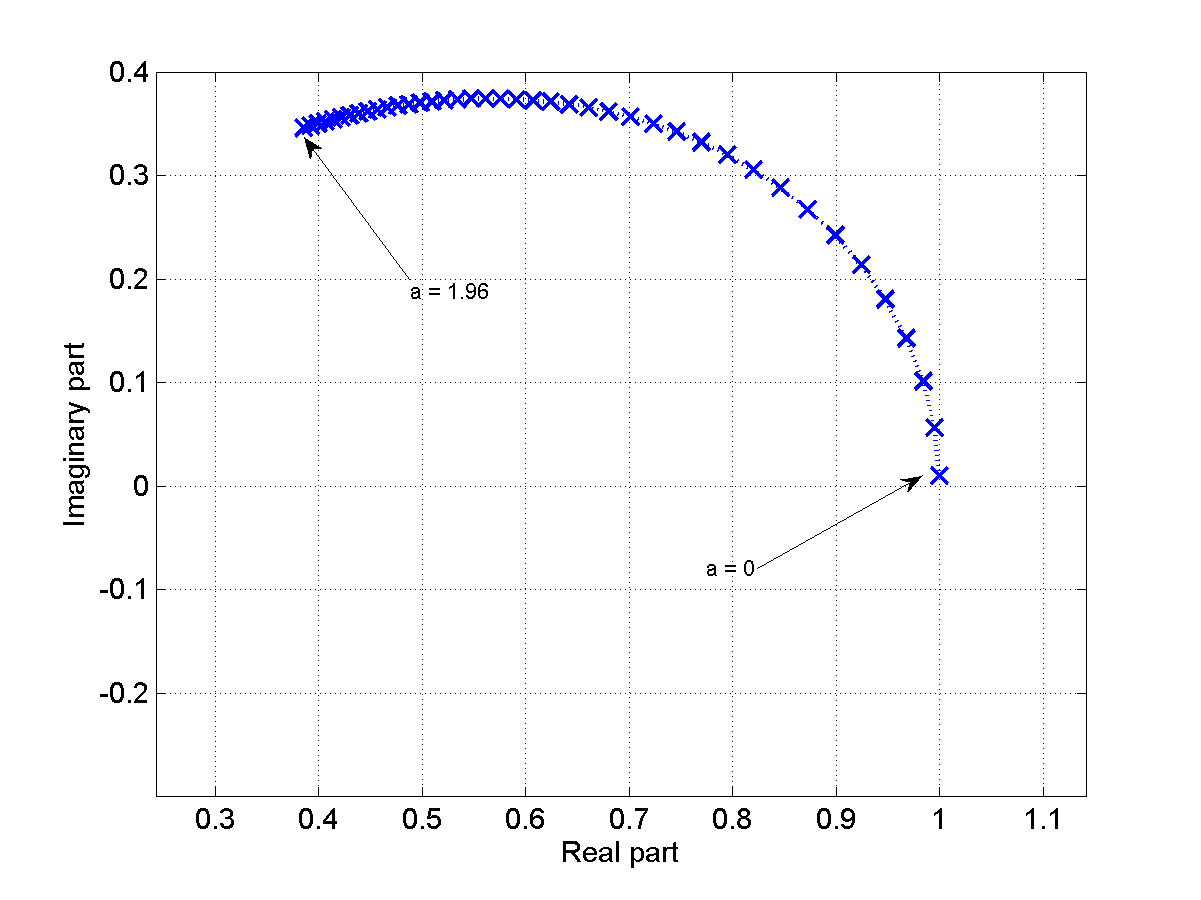It does not seem to be a circle. This is purely empirical, I have no clue if/how one could find these values analytically (but this would be very nice).

While playing with these numbers I realized that my original observation that one would always converge to one fixed point regardless of the initialization was actually wrong. For some points, the series diverged to infinity. This sparked the question where these points would be located on the complex plane. So I made a second set of experiments: I started the series from initial points $x_0$ chosen in a regular grid in the complex plane, let the series run for a predefined maximum number of iterations and observed what it does: (a) does it diverge to infinity or (b) does it converge to the fixed points I found earlier (i.e., get within a predefined threshold $\delta$ of it)?

I began with $\alpha = j\cdot \pi/2$ (corresponding to the $x_{n+1} = j^x_{n}$ it all started with) and a cartesian grid in ${\rm Re}(x_0)$ and ${\rm Im}(x_0)$ for the initial points. And then, I got this: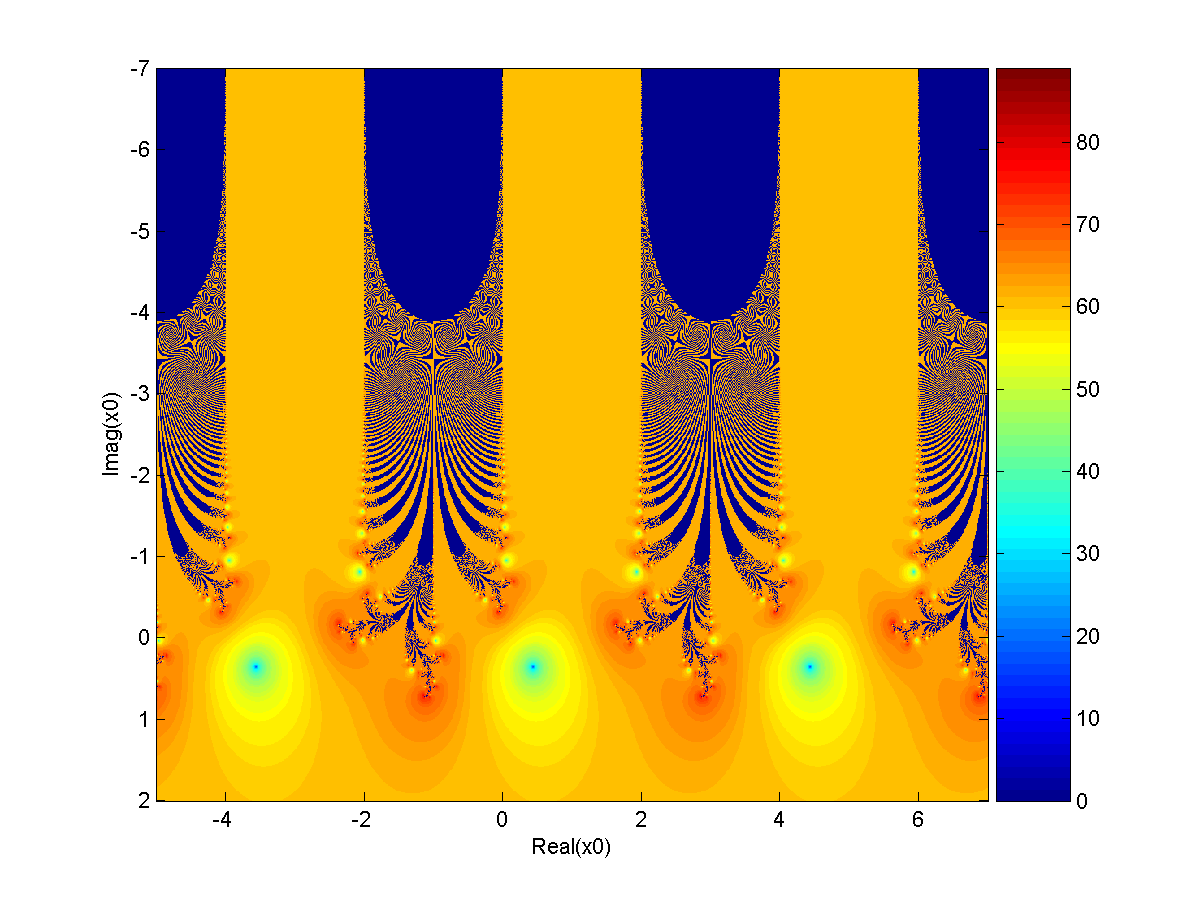The two axis refer to the real part and the imaginary part of our initial point $x_0$. The color indicated the number of iterations until the fixed point is reached up to an accuracy of $10^{-3}$. We notice the periodicity in ${\rm Re}(x_0)$: this is not surprising since the first step in the series gives

$x_1 = {\rm e}^{j \cdot \pi/2 \cdot x_0} = {\rm e}^{-\pi/2 \cdot {\rm Im}(x_0)} \cdot {\rm e}^{j \cdot \pi/2 \cdot {\rm Re}(x_0)}$

and therefore, adding an integer multiple of 4 to ${\rm Re}(x_0)$ gives the same $x_1$. I found the shape looks very interesting and started zooming in.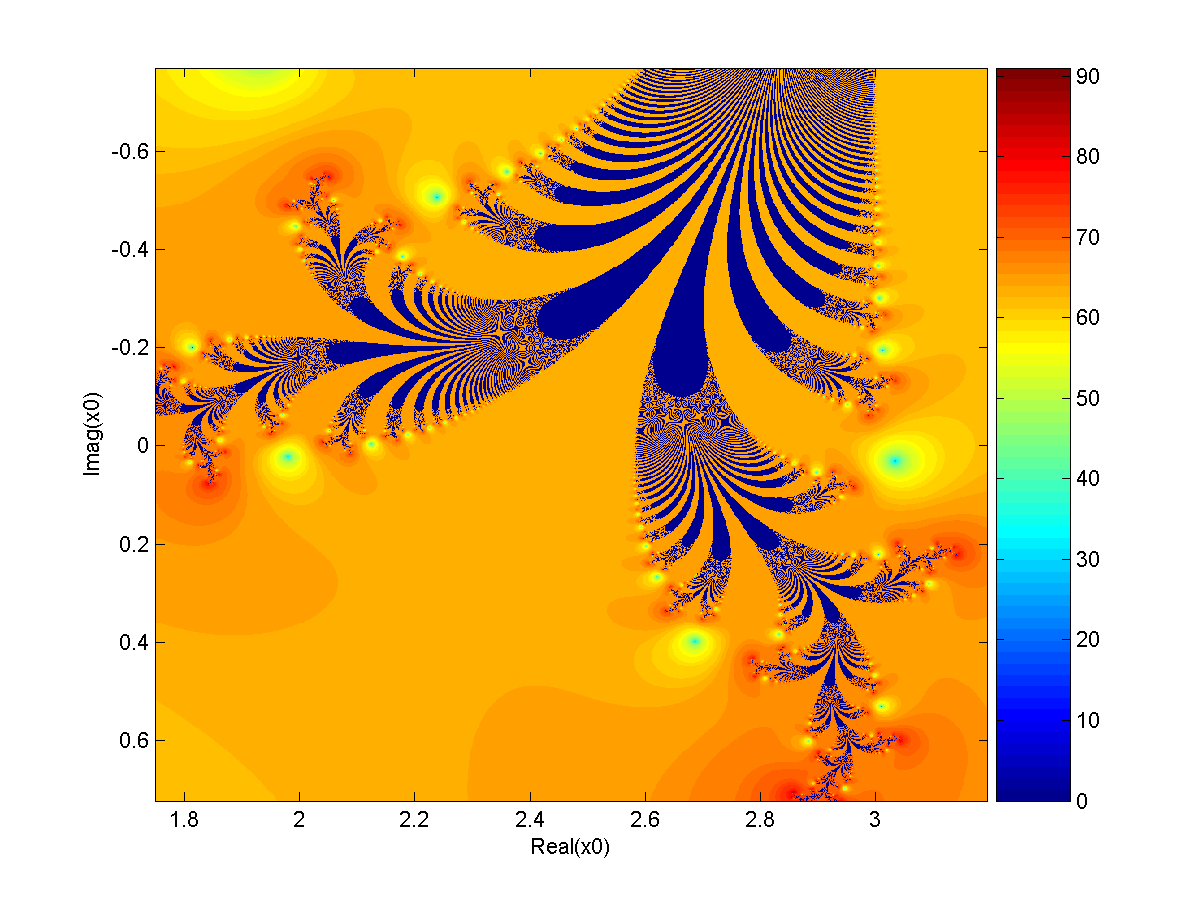Even further…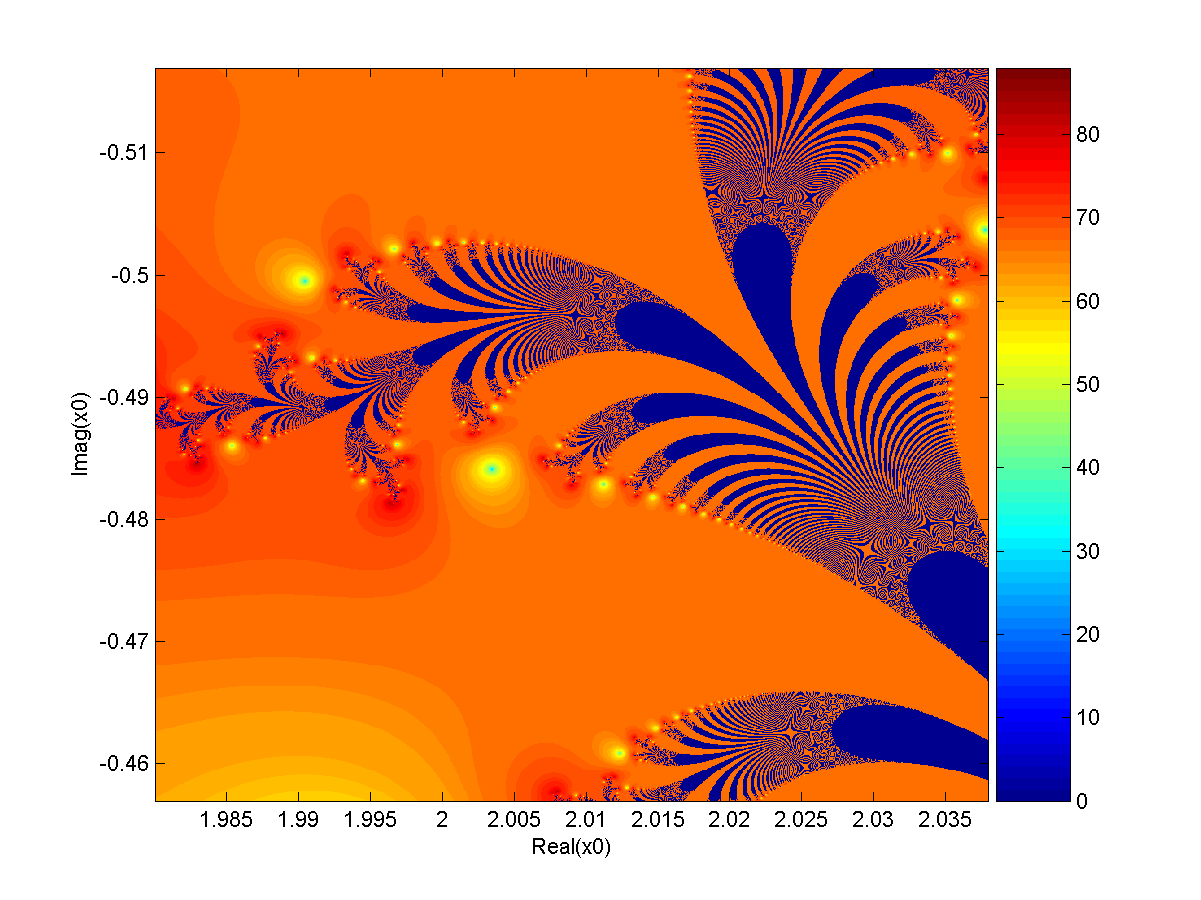This is already a 200x zoom on both axis compared to the original one. Yet more and more details are revealed that look similar to the original ones – we seem to have self-similarity here. I’m not sure if that’s enough to call this a fractal but I’d like to claim so until someone convinces me otherwise.

I have since played with various values of $\alpha$, kept zooming in and looking at the result. So far it seems that the level of details keeps increasing as $\alpha$ approaches $2 \cdot j$. After about $1.961 \cdot j$ it becomes hard to still draw a picture because instead of unique attractors the fractal seems to run into limit cycles consisting of a discrete number of points. There still seems to be a fractal-looking region of divergence but I’m mostly guessing here.

Here is one  for $\alpha = j \cdot 1.96$: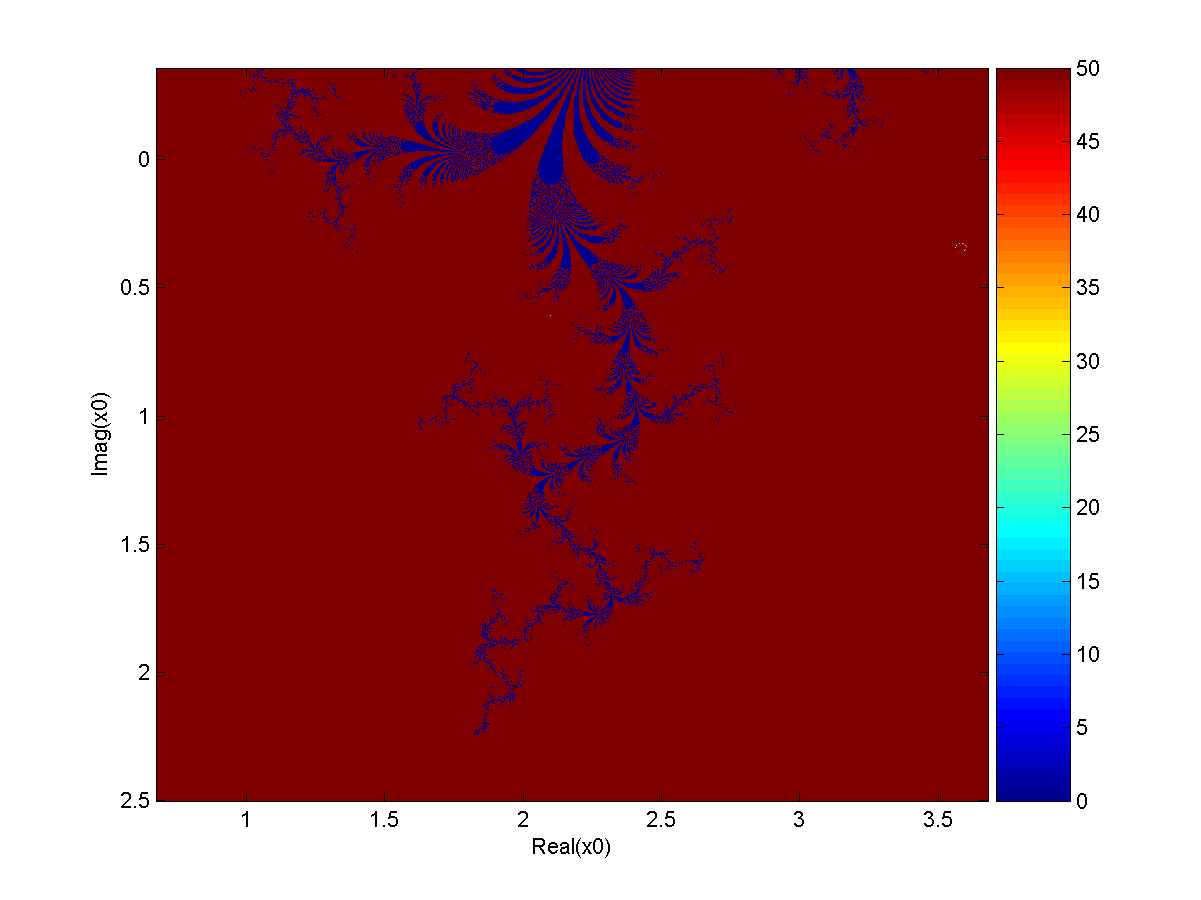I chose 50 as the maximum number of iterations here, the picture does not change much if I increase it to 1000 or so (it just takes much longer to render).

Here is my best attempt for $\alpha = j \cdot 1.963495$:I display the plane of initial points in polar instead of cartesian coordinates (for cosmetic reasons only) so this one is periodic in the vertical direction and truncated on the right side horizontally. The maximum number of iterations was set to 2000 here, increasing it further does have an impact on the larger red areas that get filled more and more. I tried to count how long it takes until a limiting cycle is reached but this is based on very rough heuristics and might be wrong. I still enjoy the look of the result.

It’s really a lot of fun to “explore” this little world, especially if you’ve never seen it before and you’re not sure if anyone else ever has. It’s quite possible these fractals are actually well-known, I just don’t know what I should search for. In light of this it would be really great if anyone could give me any hint that brings me closer to any of these open questions:

• Is this class of series known, does it have a name, is there any published material on it?
• How can we compute the fixed points of the series for a given value of $\alpha$?
• Are the resulting images that divide converging from diverging initial points actually a fractal? Does it possibly also have a name, has it been studied before?
• What happens beyond the “magic” breaking point where the series starts running into limit cycles comprising of a discrete number of points? Does the breaking point have any special meaning? Can the limit cycles be described analytically?

If you have any idea to any of these please leave me a comment!

## A funny number

I was giving tutorials for an undergraduate course in Signals and Systems theory. Since students came from quite varying backgrounds I had to start very simple – my first tutorial used to be just on complex numbers. This left some of the students bored, saying they’ve seen it all. To keep them thinking I usually asked them to compute a complex number for me: Let $j$ be the imaginary unit, what is the value of $j^j$?

This question is not as trivial as it may seem at first sight. It requires a generalization of the exponentiation $a^b$ from $a, b \in \mathbb{R}$ to $a, b \in \mathbb{C}$. There are rigorous ways of doing this which I do not want to discuss in detail here. Let us just assume that we found a generalization of $a^b$ to complex numbers which satisfies the laws of powers, in particular the law $a^b = {\rm e}^{b \cdot \ln(a)}$ where $\ln(x)$ is the natural (base-e) logarithm. Then, we can rewrite $j^j$ as $j^j = {\rm e}^{j \cdot \ln(j)}$. What is $\ln(j)$ though? Well, $j$ can be written as ${\rm e}^{j \cdot \pi/2}$, so $\ln(j)$ should be $j \cdot \pi/2$, right? This finally brings us to $j^j = {\rm e}^{j \cdot j \cdot \pi/2} = {\rm e}^{-\pi/2}$.

Some of my students would actually obtain this result. They would usually be surprised to get a real (and sort of strange number) out of such an operation, but always they would be very proud to have the answer. The tech-savvy ones would even check their result with whatever internet-able device they carried and be extra sure to have it right.

However, my (admittedly a bit discouraging) reply would be that the answer is, despite being correct, incomplete. Actually infinitely incomplete. There are (infinitely) more “correct” answers. How so? Well, $j$ can be written as ${\rm e}^{j \cdot \pi/2}$ but it can also be written as ${\rm e}^{j \cdot (\pi/2 + 2\pi)}$ or ${\rm e}^{j \cdot (\pi/2 – 2\pi)}$, and so on. We can add any integer multiple of $2\pi$ to the phase due to the periodicity of complex numbers with respect to their phase.

For the (natural) logarithm of a complex number this means that it is in general ambiguous: $\ln(j) = j \cdot \pi/2 + 2\cdot k \cdot \pi$ for any $k \in \mathbb{Z}$. To avoid the confusion it is common to write ${\rm Ln}(x) = \ln(x) + 2\cdot k \cdot \pi$ where $\ln(x)$ is the (unique) principle value of the logarithm which we obtain by choosing the principle phase of $x$.

In that sense, the full answer would be $j^j = {\rm e}^{-\pi/2} \cdot {\rm e}^{-2 \cdot k \cdot \pi}$, where $k=0$ corresponds to the principle value of $j^j$ given by ${\rm e}^{-\pi/2}$.

Infinitely many solutions, all of them are real, and all of them connect ${\rm e}$ and $\pi$. Pretty cool, right?

## Finally

Finally, I managed to update the site a bit and to put my dissertation online. You can now find it on the main site unter “Dissertation”, here is a link for you.

I’d be very happy to hear your feedback. I hope you will enjoy reading it.

## Long time no post…

… I apologize. But I have a good excuse: I was really really busy trying to finish my thesis. That’s right folks, this guy has submitted his dissertation recently and the last weeks towards this goal were quite … intense.

I’m not entirely happy with the result but that’s normal I think, noone ever is. Still I’m happy I can again focus on other things in life.

I will make the thesis publicly available as soon as I am officially allowed to do so, likely some time in October. I’ve decided to publish it under a Creative Commons BY-NC-ND license, so you will be free to copy/print/read it as often as you like. 😉

Until then, stay tuned and keep your fingers crossed for the defense that I still have to go through. 😉